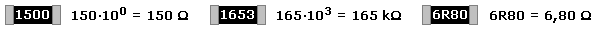# How big is a 0201 resistance

## Coding for SMD resistors

The SMD resistors, surface-mounted components, were initially only available as MELF resistors (Metal Electrode Faces). They were still big enough to show their value through the familiar color ring code. Further miniaturization led to the actual SMD chips and a new coding. The function must also be clearly identifiable from it, since it cannot always be recognized on the SMD chip. A chip with two contacts could be a resistor, capacitor, inductor, or diode. SMD resistors are identified with two, three and four-digit combinations of letters and numbers. Very small SMD chips 0603 (L x W = 1.5 x 0.8 in mm) are sometimes not labeled. For even smaller designs from 0402 (1 x 0.5 in mm) there is no longer any space for a label.

Two-digit character code

The identifier consists of a letter followed by a number. The capital letters correspond to the value from the 1st decade of the E24 standard series with a tolerance of ± 5%. If the code begins with a lowercase letter, it is a special value from 2.5 in a different gradation. The following equation is used to calculate the standard resistance values ​​of the E24 series rounded to one decimal place. The two-character SMD code can be deciphered using the table.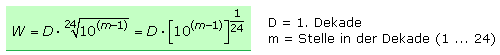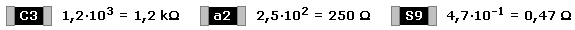Three-digit code

The resistance values ​​correspond to the standard series E24 with a tolerance of ± 5%. The manufacturing process often delivers more precise values ​​with a tolerance of ± 1%. The numerical value of the first two digits is to be multiplied by the power of ten of the third digit. If the middle digit is underlined, the best possible tolerance is ± 5% or worse. The table shows the assignment.

The number for the tens exponent can be missing. The coding then consists of two digits and the letter R, which is a decimal point. The resistance value must be given in ohms. Here are some examples of the 3-digit code.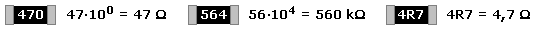Three-digit character code

The identifier is made up of two digits that stand for a three-digit value. The key character for the multiplier follows as a capital letter. The resistance values ​​correspond to the standard series E96 with a tolerance of ± 1%. The table can be used to determine the value from the three-digit character code.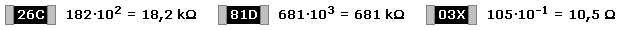Four-digit code

The first three digits indicate the direct numerical value and the fourth digit the tens exponent of the multiplier. The last digit can also be interpreted as the number of zeros to be added. The coding corresponds to the color code of the E96 series with a tolerance of ± 1% for wired resistors. If there is an R in the digit code, then it is at the decimal point of the resistance value in ohms and the multiplier is missing.